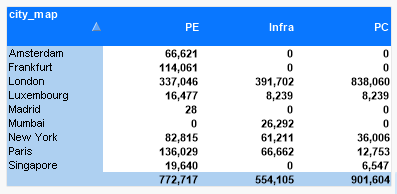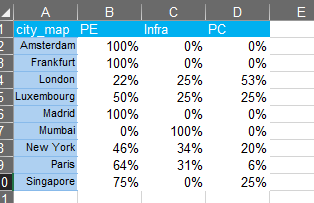# QlikView App Dev

Discussion Board for collaboration related to QlikView App Development.

Announcements
cancel
Showing results for
Did you mean:Creator III

## Set analysis: sum aggr

Hello

I have a chart shown below. I would like to get some set analysis code that I can use in my N Printing report for the totals , eg 772,717 and 554,105 and 901,604The expression eg for the PE column is

sum({\$<period = {">=\$(vStartPeriod_CY)<=\$(vEndPeriod_CY)"},
costc = {681,685},
bud2_line = {"OFFICE COSTS"},
Datasource = {'Actual'}
>}
\$(vCurrency)) * PE%

PE% is a percentage held in a spreadsheet that I load into QV

When I take this code and put it into a text box it doesn't work - it brings back zero, even though it works in the table.

I wondered if it is anything to do with aggregating by city_map

I tried the below but this although it brings back a number it brings back the wrong number - can you suggest what I am doing wrong?

=Sum(Aggr(

sum({\$<period = {">=\$(vStartPeriod_CY)<=\$(vEndPeriod_CY)"},
bud2 = {"200"},
costc = {681,685},
bud2_line = {"OFFICE COSTS"},
Datasource = {'Actual'}
>}
\$(vCurrency))
*
PE%
,
city_map))

Many thanks

Paul

5 RepliesMVP

Try by adding the inner aggregation's set analysis to the outer aggregation

=Sum({\$<period = {">=\$(vStartPeriod_CY)<=\$(vEndPeriod_CY)"}, bud2 = {"200"}, costc = {681,685}, bud2_line = {"OFFICE COSTS"}, Datasource = {'Actual'}>} Aggr(Sum({\$<period = {">=\$(vStartPeriod_CY)<=\$(vEndPeriod_CY)"}, bud2 = {"200"}, costc = {681,685}, bud2_line = {"OFFICE COSTS"}, Datasource = {'Actual'}>} \$(vCurrency)) * PE%, city_map))Creator III
Author

Thanks Sunny

That gave me the same answer as I was getting.

If I use this code, ie take off the final *PE%  (and for the other columns it was *INF% and *PC% then I get the total of the 3 columns, which would be correct

But when I use the *PE% in a text field I get the wrong result in the PE column (and the Infra/PC columns)

sum({\$<period = {">=\$(vStartPeriod_CY)<=\$(vEndPeriod_CY)"},
costc = {681,685},
bud2_line = {"OFFICE COSTS"},

Datasource = {'Actual'}
>}
\$(vCurrency)Creator III
Author

..just to add each city_map has a different %ge that it is multiplied by ie that's why I thought you would aggregate somehowMVP

There are two dimensions in the chart? Add both to your Aggr() functionCreator III
Author

Thanks Sunny

Not sure why this now works but this worked for me

=Sum(Aggr(
sum({\$<period = {">=\$(vStartPeriod_CY)<=\$(vEndPeriod_CY)"},
costc = {681,685},
bud2_line = {"OFFICE COSTS"},

Datasource = {'Actual'}
>}
\$(vCurrency)) * PE%
,
PE%))